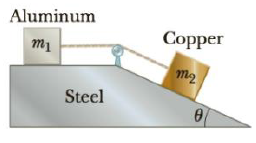Chapter 4, Problem 83AP

Chapter
Section
Textbook Problem

A 2.00-kg aluminum block and a 6.00-kg copper block are connected by a light string over a frictionless pulley. The two blocks are allowed to move on a fixed steel block wedge (of angle θ = 30.0°) as shown in Figure P4.83. Making use of Table 4.2, determine (a) the acceleration of the two blocks and (b) the tension in the string.Figure P4.83

(a)

To determine
The acceleration of the blocks.

Explanation

Given info: Mass of aluminum block is 2.00 kg. Mass of copper block is 6.00 kg. Co-efficient of friction for aluminum and copper are 0.47 and 0.36 respectively.

The free body diagram is given below.

• N1 and N2 are the normal forces.
• ma and mc are the masse of the aluminum and copper blocks.
• g is the acceleration due to gravity.
• f1 and f2 are the friction forces.

From the diagram,

For the Aluminum block,

Tf1=maa (I)

N1=mag (II)

For the Copper block,

mcgsin30οTf2=mca (III)

N2=mcgcos30ο (IV)

The formula for the friction forces is,

f1=μaN1 (V)

f2=μcmcgcos30ο (VI)

• μa and μc are the co-efficient of friction for Aluminum and Copper respectively.

Substitute Equations (II) and (IV) in (V) and (VI) respectively.

f1=μamag

f2=μcmcg

Substitute the above equations in (I) and (III) respectively.

Tμamag=maa (VII)

mcgsin30οTμcmcgcos30ο=mca (VIII)

Add Equation (VII) and (VIII) and re-arrange to get a

(b)

To determine
The tension in the string.

Still sussing out bartleby?

Check out a sample textbook solution.

See a sample solution

The Solution to Your Study Problems

Bartleby provides explanations to thousands of textbook problems written by our experts, many with advanced degrees!

Get Started